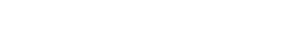# Set Language And Absolute Value | Cambridge IGCSE MathsThe Cambridge IGCSE mathematics curriculum consists of the concepts of sets and absolute value. Therefore, it is important to be well-versed in these concepts if you are sitting for the Cambridge IGCSE Mathematics examination. Please view the video at the end of this blog post for a visual explanation of the following concepts and practice questions.

## Set Language And Notations Of Venn Diagrams

Set language is a language that is used to define sets and describe relationships between different types of sets in mathematics. The set language is based on mathematical symbols and is used to denote various mathematical concepts like functions and relations.

In simple terms, sets are used to organize and group things together in mathematics. You can think of sets as a container holding many objects. These objects are known as elements or numbers of the set. It is important to note that the elements in a set should be unique, you can’t have the same element in the same set twice.

Different types of sets and their relationships are visually represented via Venn diagrams. The notation in Venn diagrams includes overlapping circles that represent sets and the area of overlap between the circles represents the relationship between the sets.

Let’s take a look at a few common notations used in Venn diagrams:

Union

The union of two sets is a set that contains elements from both sets. In Venn diagrams, it is represented by the whole area enclosed by the two circles including the area the circles overlap. The symbol that denotes a union is ‘U’.

For example, if  A and B are two sets, the union of A and B is the new set or area that contains elements from both A and B plus the elements in each individual set. As in the snippet below, the union of A and B is the whole area that’s highlighted in green.Intersection

The intersection of two sets in a Venn diagram is the area that has only the elements common to both sets. The symbol of an intersection is denoted by an upside-down ‘U’ –  ‘n’.

For example, if A and B are two sets, the intersection of A and B is the area that contains only elements from both sets A and B. As in the snippet below, the intersection of A and B is the area highlighted in green.Subset

The subset in a Venn diagram is a set of elements within another set. In a Venn diagram, this is typically represented by a circle that is completely contained within another circle. In simple terms, the smaller circle denotes the subset and the bigger circle represents the set that contains the subset. The symbol that denotes a subset is a slightly long c [refer to the image below].

For example, if A and B are two sets and if A is contained within B, we can say that set A is a subset of set B. Please refer below for a visual representation of this:Member / Element

The member symbol is also known as an ‘element’ and it represents an individual element that is part of a set. This notation is denoted by an E with a line through it – it’s very similar to the Euro currency sign.

For example, if we have a set X that contains a, b, c, and d, we can say that a is a member of X, b is a member of X, C, is a member of X, and so on. The snippet below shows a visual representation of this.Complement

The complement notation is denoted by an apostrophe sign (‘).

This notation basically represents the elements that are not part of a given set. For example, if we have a set ‘A’ that contains the items a,b,c, and d, the complement of set A are the elements that are not in set A.

In the following snippet, the complement of A [ A’ ] is the area that is shaded in green.Universal Set

The universal set is defined as the set that contains all the elements being considered in a particular problem. For example, if we are comparing the sets ‘cars’ and ‘buses’, the universal set would be ‘all vehicles’.

In Venn diagrams, a universal set is represented by a large rectangle or square that contains all circles representing the sets being compared. Refer to the following image for a visual representation of this:Number of / Number of Elements

This notation is used to represent the number of elements in a given set. The symbol that is used to denote this is n (A).Empty Set

An empty set is a set that contains no elements. This is generally represented by a circle with a diagonal line drawn through it.## Absolute Value

The absolute value of a number is a value that represents the positive value of a number [the non-negative value]. In simple terms, the absolute value of a positive number is equal to the number itself and the absolute value of a negative number is equal to the positive value of that same number.

For example, the absolute value of 6 is 6 and the absolute value of – 6 is also 6.The key to successfully passing your Cambridge IGCSE Maths exams is to practice thoroughly under exam conditions. Tutopiya’s online learning platform is designed especially for this purpose. You can study this particular sub-topic using our resources and thereafter attempt the quiz on our question bank to see how well you do. Good luck!